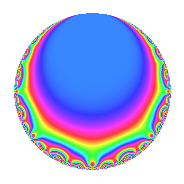# Properties

 Label 336.2.b.aLevel 336 Weight 2 Character orbit 336.b Analytic conductor 2.683 Analytic rank 0 Dimension 2 CM No Inner twists 2

# Related objects

## Newspace parameters

 Level: $$N$$ = $$336 = 2^{4} \cdot 3 \cdot 7$$ Weight: $$k$$ = $$2$$ Character orbit: $$[\chi]$$ = 336.b (of order $$2$$ and degree $$1$$)

## Newform invariants

 Self dual: No Analytic conductor: $$2.68297350792$$ Analytic rank: $$0$$ Dimension: $$2$$ Coefficient field: $$\Q(\sqrt{-3})$$ Coefficient ring: $$\Z[a_1, \ldots, a_{7}]$$ Coefficient ring index: $$2$$ Sato-Tate group: $\mathrm{SU}(2)[C_{2}]$

## $q$-expansion

Coefficients of the $$q$$-expansion are expressed in terms of a primitive root of unity $$\zeta_{6}$$. We also show the integral $$q$$-expansion of the trace form.

 $$f(q)$$ $$=$$ $$q - q^{3} + ( -1 - 2 \zeta_{6} ) q^{7} + q^{9} +O(q^{10})$$ $$q - q^{3} + ( -1 - 2 \zeta_{6} ) q^{7} + q^{9} + ( 2 - 4 \zeta_{6} ) q^{11} + ( 4 - 8 \zeta_{6} ) q^{17} -4 q^{19} + ( 1 + 2 \zeta_{6} ) q^{21} + ( 2 - 4 \zeta_{6} ) q^{23} + 5 q^{25} - q^{27} -6 q^{29} + 4 q^{31} + ( -2 + 4 \zeta_{6} ) q^{33} -2 q^{37} + ( 4 - 8 \zeta_{6} ) q^{41} + ( -2 + 4 \zeta_{6} ) q^{43} + ( -3 + 8 \zeta_{6} ) q^{49} + ( -4 + 8 \zeta_{6} ) q^{51} -6 q^{53} + 4 q^{57} + 12 q^{59} + ( -8 + 16 \zeta_{6} ) q^{61} + ( -1 - 2 \zeta_{6} ) q^{63} + ( -2 + 4 \zeta_{6} ) q^{67} + ( -2 + 4 \zeta_{6} ) q^{69} + ( -6 + 12 \zeta_{6} ) q^{71} + ( 8 - 16 \zeta_{6} ) q^{73} -5 q^{75} + ( -10 + 8 \zeta_{6} ) q^{77} + ( -6 + 12 \zeta_{6} ) q^{79} + q^{81} + 12 q^{83} + 6 q^{87} + ( -4 + 8 \zeta_{6} ) q^{89} -4 q^{93} + ( 2 - 4 \zeta_{6} ) q^{99} +O(q^{100})$$ $$\operatorname{Tr}(f)(q)$$ $$=$$ $$2q - 2q^{3} - 4q^{7} + 2q^{9} + O(q^{10})$$ $$2q - 2q^{3} - 4q^{7} + 2q^{9} - 8q^{19} + 4q^{21} + 10q^{25} - 2q^{27} - 12q^{29} + 8q^{31} - 4q^{37} + 2q^{49} - 12q^{53} + 8q^{57} + 24q^{59} - 4q^{63} - 10q^{75} - 12q^{77} + 2q^{81} + 24q^{83} + 12q^{87} - 8q^{93} + O(q^{100})$$

## Character Values

We give the values of $$\chi$$ on generators for $$\left(\mathbb{Z}/336\mathbb{Z}\right)^\times$$.

 $$n$$ $$85$$ $$113$$ $$127$$ $$241$$ $$\chi(n)$$ $$1$$ $$1$$ $$-1$$ $$-1$$

## Embeddings

For each embedding $$\iota_m$$ of the coefficient field, the values $$\iota_m(a_n)$$ are shown below.

For more information on an embedded modular form you can click on its label.

Label $$\iota_m(\nu)$$ $$a_{2}$$ $$a_{3}$$ $$a_{4}$$ $$a_{5}$$ $$a_{6}$$ $$a_{7}$$ $$a_{8}$$ $$a_{9}$$ $$a_{10}$$
223.1
 0.5 + 0.866025i 0.5 − 0.866025i
0 −1.00000 0 0 0 −2.00000 1.73205i 0 1.00000 0
223.2 0 −1.00000 0 0 0 −2.00000 + 1.73205i 0 1.00000 0
 $$n$$: e.g. 2-40 or 990-1000 Significant digits: Format: Complex embeddings Normalized embeddings Satake parameters Satake angles

## Inner twists

Char. orbit Parity Mult. Self Twist Proved
1.a Even 1 trivial yes
28.d Even 1 yes

## Hecke kernels

This newform can be constructed as the intersection of the kernels of the following linear operators acting on $$S_{2}^{\mathrm{new}}(336, [\chi])$$:

 $$T_{5}$$ $$T_{19} + 4$$